# Target Area Extraction Algorithm of Infrared Thermal Image Combining Target Detection with Matching Correction

Target Area Extraction Algorithm of Infrared Thermal Image Combining Target Detection with Matching Correction
School of Software and Big Data, Changzhou College of Information Technology, Changzhou 213164, China

Corresponding Author Email:
yangdan@czcit.edu.cn
Page:
227-234
|
DOI:
https://doi.org/10.18280/ts.400121
11 December 2022
|
Accepted:
6 February 2023
|
Published:
28 February 2023
| Citation

OPEN ACCESS

Abstract:

Infrared thermal image makes the target have certain degree of recognition by reflecting the thermal radiation information emitted by the target, which effectively compensates the information loss of visible light image in harsh imaging environment. Contour extraction effect of target area using traditional Canny algorithm is not good, because the contour gradient change of infrared thermal image target area is not obvious. At the same time, the threshold of most of algorithms needs to be set manually, which is greatly affected by subjective factors, and the image processing efficiency is low. Therefore, this paper studied the target area extraction algorithm of infrared thermal image by combining target detection with matching correction. First, the paper introduced the feature matching algorithm based on grid motion statistics, and converted smoothness constraint of motion into statistics, thus replacing the number of extended feature points with the acquisition of features with better performance and filtering false image matching based on the number of other matching points in the neighborhood of statistical matching points. Second, based on the feature matching results obtained in the previous section, this paper proposed a extraction method of infrared thermal image target area based on thermal feature descriptors, which combined the extracted thermal features with the semantic attributes of each area in the infrared thermal image, thus distinguishing the subtle differences between the infrared thermal image sub-categories. Finally, experimental results verified the effectiveness of the proposed method.

Keywords:

target detection, image matching, infrared thermal image, target area extraction

1. Introduction

Target detection and target area extraction are important research contents in the image processing field, which are widely used in security monitoring, battlefield environment awareness, unmanned driving and other scenes [1-11]. However, the commonly used industrial camera lost a lot of useful information when collecting images in extreme environmental conditions, such as darkness and haze. Infrared thermal image obtained using thermal infrared detection technology made the target have certain degree of recognition by reflecting the thermal radiation information emitted by the target, effectively compensating the information loss of visible light image in harsh imaging environment [12-23]. Therefore, the research on the target area extraction algorithm of infrared thermal image is very important for improving the detection robustness and accuracy of interested targets in harsh environment.

Fusion analysis of thermal infrared image and visible image has been widely used in intelligent security and fault detection. For the difficulty in matching two images in the fusion process in the same scene, Zhi et al.  proposed an image registration method based on feature contour quadrilateral. First, segmentation algorithm was used to filter the thermal infrared image and the visible image respectively. Second, the study detected contour points and redrew the contours. Then the algorithm was used to filter out the feature contours and approximate the polygon, thus generating the minimum circumscribed quadrilateral of all contours. Experimental results showed that this method effectively reduced the matching error and made the contour positioning more accurate, thus achieving high-precision and rapid image registration. Akula et al.  introduced WignerMSER, which is both a robust local feature detector of thermal infrared image, and a new variant of the famous Maximally Stable Extremal Regions (MSER) feature detector. The proposed WignerMSER feature was obtained by transforming the image from spatial domain to joint spatial frequency domain using the pseudo Wigner-Ville distribution, and then by detecting the MSER in Wigner transform space. The overall classification accuracy of the fused WignerMSER feature detector on two data sets increased more than 5% than that of the traditional MSER, showing the best performance. Zhang et al.  proposed a method for screening the zero-value insulator infrared thermal image features based on binary logical regression analysis. The study first de-noised the infrared thermal image by combining wavelet transform with mean filtering, and enhanced the contrast of image using the histogram equalization method. Experiment result showed that the proposed selection method was simple and effectively screened feature parameters. Features needed to be extracted from infrared thermal sequence data in order to visually display defect features. Because different types of pixels in the damaged area were stimulated differently by heat after temperature change, the improved mean shift clustering algorithm relied on the pixels in space to obtain transient thermal response (TTR) with different types of damage according to the TTR change trend of pixels. Lei et al.  adjusted various damaged focal points on the selected typical TTR using the dynamic weighted multi-objective optimization adjustment decomposition algorithm.

Two steps of target detection and matching correction needed to be achieved in order to completely extract the target area of specific target in infrared thermal image. The existing research methods at home and abroad cannot realize the pattern matching of simple infrared thermal image through automatic learning of a large number of samples. Effect of extracting target area contours using traditional Canny algorithm is not good, because there is no obvious contour gradient change in the infrared thermal image target area. At the same time, the threshold of most of algorithms needs to be set manually, which is greatly affected by subjective factors, and the image processing efficiency is low. Therefore, this paper studied the target area extraction algorithm of infrared thermal image by combining target detection with matching correction. Chapter 2 introduced the feature matching algorithm based on grid motion statistics, and converted smoothness constraint of motion into statistics, thus replacing the number of extended feature points with the acquisition of features with better performance. In addition, false image matching was filtered based on the number of other matching points in the neighborhood of statistical matching points. Based on the feature matching results obtained in the previous section, Chapter 3 proposed a method extracting infrared thermal image target area based on thermal feature descriptors, combining the extracted thermal features with the semantic attributes of all areas in the image, thus distinguishing the subtle differences between the infrared thermal image sub-categories. Finally, experimental results verified that the proposed method was effective.

2. Feature Matching of Infrared Thermal Image Based on Grid Statistics

## 1.png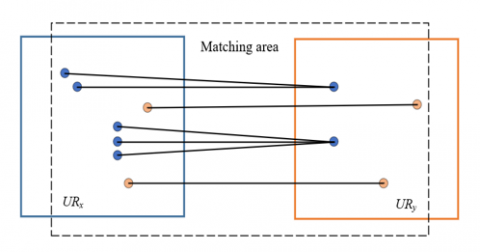Figure 1. Feature points in the matching area

## 2.png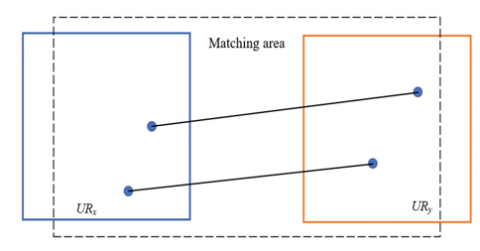Figure 2. Correct matching points in the matching area

In this chapter, feature matching algorithm based on grid motion statistics was used for target detection and matching correction of infrared thermal image target area. Different from traditional artificial feature detection algorithm, the adopted algorithm converted the smoothness constraint of motion into statistics, replaced the number of extended feature points with the acquisition of features with better performance, and filtered out false image matching based on the number of other matching points in the neighborhood of the statistical matching points. The algorithm did not rely on prior knowledge of the designer and had a wide range of application and good performance in mining deep features of the image.

Let URx be the reference image, URy be the image to be matched, URx and URy contain N and M feature points, respectively, and let {N, M} be the corresponding feature point set. Figure 1 and Figure 2 show feature points and correctly matched feature points in the matching area. a={a1,a2, ... ,aN} represented the matching point pair set, obtained by matching {N, M} based on BF algorithm. True and false matching in a={a1,a2, ... ,aN} was recognized by analyzing the support matching pair in the neighborhood of each matching point in {N, M}. Let Ri score be the support estimator of matching points in a neighborhood, and ψi be the number of matching point pairs in the corresponding area, then there was:

${{R}_{i}}=\left| {{\psi }_{i}}-1 \right|$                        (1)

It’s assumed that area x has n support feature points, with gx being one of them. Let o be the correct matching probability corresponding to gx, Oxy and Gxy be the correct and false matching of area x and area y, respectively, gyx be the matching point corresponding to gx within area y, g'yx be the matching point corresponding to gx outside area y, and gox and ggx be the correct and false matching of feature points in gx and gy, respectively.

Let M be the total number of feature points in the image to be matched, γ be the regulation parameter, and n be the number of feature points in area y. When gx was falsely matched, the probability of its matching point in area y was calculated by the following formula:

$y\left( g_{x}^{y}|g_{x}^{g} \right)=\frac{\gamma m}{M};\gamma \in \left( 0,1 \right)$                          (2)

When the area {x, y} corresponded to the same location, the probability Eo of the nearest neighbor matching of gx in area y was calculated based on the following formula:

\begin{align} & {{E}_{o}}=e\left( g_{x}^{y}|{{O}^{xy}} \right)=e\left( g_{x}^{o}|{{O}^{xy}} \right)+e\left( g_{x}^{g},g_{x}^{y}|{{O}^{xy}} \right) \\ & =e\left( g_{x}^{o}|{{O}^{xy}} \right)+e\left( g_{x}^{g}|{{O}^{xy}} \right)e\left( g_{x}^{y}|g_{x}^{g},{{O}^{xy}} \right) \\ & =e\left( g_{x}^{o} \right)+e\left( g_{x}^{g} \right)e\left( g_{x}^{y}|g_{x}^{g} \right) \\ & =o+\left( 1-o \right)\gamma m/M \\\end{align}                               (3)

When the area {x, y} corresponded to different locations, the probability Eg of the nearest matching point of gx in area y was calculated based on the following formula:

\begin{align} & {{E}_{g}}=e\left( g_{x}^{y}|{{G}^{xy}} \right) \\ & =e\left( g_{x}^{g},g_{x}^{y}|{{G}^{xy}} \right) \\ & =e\left( g_{x}^{g}|{{G}^{xy}} \right)e\left( g_{x}^{y}|g_{x}^{g},{{G}^{xy}} \right) \\ & =e\left( g_{x}^{g} \right)e\left( g_{x}^{y}|g_{x}^{g} \right) \\ & =\gamma \left( \text{1-}o \right)\left( m/M \right) \\\end{align}                                  (4)

Due to independence of each matching pair in the target area of infrared thermal image, the distribution of matching number R in a neighborhood was estimated through a pair of binomial distribution, based on the above calculation results:

{{R}_{i}}\sim\left\{ \begin{align} & Y\left( m,{{e}_{o}} \right),if\text{ }{{a}_{i}}\text{ }is\text{ }true \\ & Y\left( m,{{e}_{g}} \right),if\text{ }{{a}_{i}}\text{ }is\text{ }false \\\end{align} \right.      (5)

If the area to be matched was large, then there was some false matching, which inevitably affected the judgment performance of Ri score for matching results. Let L be the number of areas which moved together but did not intersect predicted by the matching point ai, and ψxlyl be the matching subset of {xl, yl} in the prediction area, then the following formula gave a more general expression of Ri:

${{R}_{i}}=\sum\limits_{l=1}^{L}{\left| {{\psi }_{{{x}^{l}}{{y}^{l}}}} \right|}-1$                 (6)

In practical application of the algorithm, it should be noted that the neighborhood did not meet the requirements of a small area. Therefore, this paper chose to divide a large area into L small areas, and there was R distribution expansion expression in the following formula:

{{R}_{i}}\sim\left\{ \begin{align} & Y\left( Lm,{{e}_{p}} \right),if\text{ }{{a}_{i}}\text{ }is\text{ }true \\ & Y\left( Lm,{{e}_{g}} \right),if\text{ }{{a}_{i}}\text{ }is\text{ }false \\\end{align} \right.           (7)

Mean value and standard deviation of R distribution were further obtained based on the following formula:

\begin{align} & \left\{ {{n}_{o}}=Lm{{e}_{o}},{{r}_{o}}=\sqrt{Lmo\left( 1-{{e}_{o}} \right)};if\text{ }{{a}_{i}}\text{ }is\text{ }true \right. \\ & \left\{ {{n}_{g}}=Lm{{e}_{g}},{{r}_{g}}=\sqrt{Lm{{e}_{g}}\left( 1-{{e}_{g}} \right)};if\text{ }{{a}_{i}}\text{ }is false \right. \\ \end{align}               (8)

Let no and ro be the mean value and standard deviation of Ri in true matching, ng and rg be the mean value and standard deviation of Ri in false matching, L be the number of neighborhoods in area x, and m be the number of feature points in the area. After introducing the distinguishing ability index E of Ri, the definition formula was as follows:

$E=\frac{{{n}_{o}}-{{n}_{g}}}{{{r}_{o}}+{{r}_{g}}}=\frac{Lm{{e}_{o}}-Lm{{e}_{g}}}{\sqrt{Lm{{e}_{o}}\left( 1-{{e}_{o}} \right)}+\sqrt{Lm{{e}_{g}}\left( 1-{{e}_{g}} \right)}}$                         (9)

Due to $E \propto(L m)^{1 / 2}$, the larger E, the better matching effect of the target area of infrared thermal image; the smaller E, the worse matching effect of the target area. Therefore, larger E was obtained by increasing L or m.

In order to make the divided area small enough to facilitate the calculation of neighborhood score Ri, this paper divided the already divided area unit into nine small grids again. Let i and j be the number of matching points of |Ailjl|, then Ri score was obtained by statistics and calculation of i and j of the matching grid pair {i,j} and the support estimators of matching points in the surrounding eight small grids.

${{R}_{ij}}=\sum\limits_{l=1}^{L=9}{\left| {{A}_{{{i}^{l}}{{j}^{l}}}} \right|}$                (10)

The following formula gave the distinction formula of true and false matching of {i, j}:

\left\{ i,j \right\}=\left\{ \begin{align} & True,if\text{ }{{R}_{ij}}>{{\phi }_{i}}=\beta \sqrt{m} \\ & False,otherwise \\\end{align} \right.                     (11)

3. Target Area Extraction of Infrared Thermal Image

In target area extraction of infrared thermal image, though a deep learning model can be constructed to extract dense local features in the image, the problem of false target area matching caused by high similarity descriptors in different local areas in the model has not been resolved and the accuracy of target area recognition is greatly affected by some incorrect matching points. Therefore, based on the feature matching results obtained in the previous section, this paper proposed a target area extraction method for infrared thermal images based on thermal feature descriptors, which combined extracted thermal features with semantic attributes of each area in the image, thus achieving the distinction of subtle differences between sub-categories of infrared thermal images. Figure 3 shows the algorithm flow.

## 3.png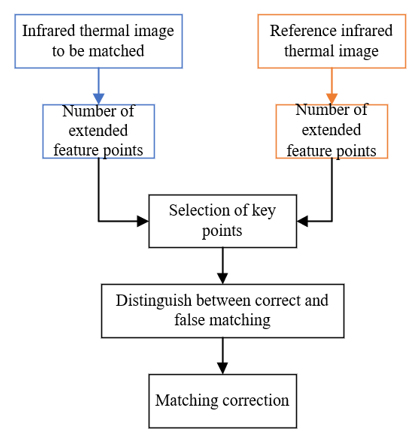Figure 3. Target area extraction process of infrared thermal image

This paper selected SegNet network as the semantic segmentation module in the target area extraction method of infrared thermal image. In order to better analyze thermal features of the target area, the SegNet network needed to be visualized in order to observe the thermal feature map of the image in specific convolution layer.

According to the visualization results, the thermal feature map, output from infrared thermal image processed by the network coding module, contained the most abundant spatial and semantic information. In order to improve the feature matching accuracy when extracting the image target area, this paper selected to obtain corresponding thermal map based on thermal feature map, thus obtaining the area where the infrared thermal image contributed the most to the output category. Let C be the size of thermal feature map, q and f be the width and height of thermal feature map, respectively, bn be the classification score, ∂bn/∂Xl be the gradient score of category n, and Xlij be the activation value of position (i,j) in the l-th channel of thermal feature map. The following gave the calculation formula for the weight of the map on prediction category m:

$\beta _{l}^{n}=\frac{1}{C}\sum\limits_{i\in q}{\sum\limits_{j\in f}{\frac{\partial {{b}^{n}}}{\partial X_{ij}^{l}}}}$                             (12)

Selection of key points in infrared thermal image was more important, because the directly extracted partial thermal features were not helpful for the target area extraction task of infrared thermal image, and brought adverse results to feature matching. Therefore, this paper trained the attention network composed of two 1×1 convolutional layers. Conv1 activation function of the network used LeaklyReLU function, which effectively reduced the vanishing gradient phenomenon in network. In addition, Conv2 activation function used SoftPlus function. The following formula gave expressions for these two functions:

$\varepsilon \left( c \right)=\left\{ \begin{matrix} \partial \times c & if\text{ }c<0 \\ c & if\text{ }c\ge 0 \\\end{matrix} \right\}$                           (13)

$g\left( a \right)=\ln \left( 1+{{t}^{a}} \right)$                            （14）

## 4.png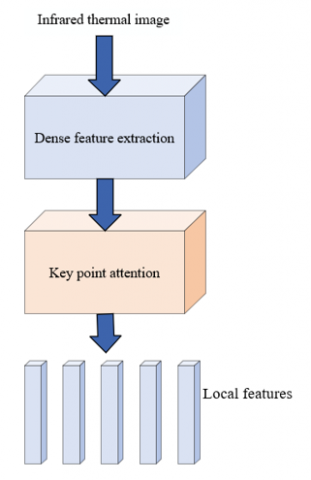Figure 4. Local feature recognition process

Attention network was used to recognize local features related to the target area. That is, the local features of infrared thermal image were ranked through attention score, and the key points required for the target area extraction task were determined through ranking results. Figure 4 shows the local feature recognition process. Figure 5 shows the execution process of attention mechanism. It’s assumed that am represents the m-th feature vector, and $a_m \in R^c$ and m=1,...M. Let c be the size of feature vector dimension, γ(am; ω) be the attention score of key points of local feature am, ω be the parameter of γ(.) function, and Q be the weight of convolutional layer connected to SoftPlus layer. The following gave the calculation formula of attention network output:

$b=Q\left( \sum\limits_{m}{\left( 1+\gamma \left( {{a}_{m}};\omega \right) \right)\times {{a}_{m}}} \right)$                    （15）

## 5.png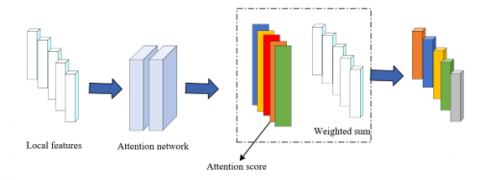Figure 5. Attention mechanism

Let b* be ground-truth in one-hot coding, and aTm be the transposition of feature vector am. The following gave the calculation formula of standard cross entropy loss function used during training:

$K=-b*\cdot \log \left( \frac{\exp \left( b \right)}{a_{m}^{T}\exp \left( b \right)} \right)$                 (16)

Back propagation training was conducted for parameter ω in γ(.), and the gradient calculation formula was given in the following formula:

$\frac{\partial K}{\partial \omega }=\frac{\partial K}{\partial b}\sum\limits_{m}{\frac{\partial b}{\partial {{\gamma }_{m}}}}\frac{\partial {{\gamma }_{m}}}{\partial \omega }=\frac{\partial K}{\partial \omega }\sum\limits_{m}{q{{g}_{m}}}\frac{\partial {{\gamma }_{m}}}{\partial \omega }$                        (17)

Because the target area feature vector of infrared thermal image extracted by the model might contain partial redundant information, the principal component analysis needed to be used to reduce dimensionality of the feature vector in order to obtain better extraction effect and faster extraction speed of the target area. The dimensionality reduction process was described in detail below.

STEP 1: L2 norm normalization was performed on the acquired target area feature. Let A=(a1,a2,...,am) be the feature vector, A2 be the normalized vector, and ||A||2 be L2 norm of vector A, then there was:

${{A}_{2}}=\left( \frac{{{a}_{1}}}{{{\left\| A \right\|}_{2}}},\frac{{{a}_{2}}}{{{\left\| A \right\|}_{2}}},..,\frac{{{a}_{m}}}{{{\left\| A \right\|}_{2}}} \right)$                       (18)

STEP 2: feature matrix AM×N with M features was represented by the following formula:

${{A}_{M\times N}}=\left[ \begin{matrix} {{a}_{1,1}} & \cdots & {{a}_{1,N}} \\ \cdots & \cdots & \cdots \\ {{a}_{M,1}} & \cdots & {{a}_{M,N}} \\\end{matrix} \right]$              (19)

The mean value of each column λi=ΣMj=1aj,i / M($i \in 1,2, \ldots, N$) of AM×N was solved. The mean value λi was subtracted by AM×N by column, and the obtained new matrix BM×N was represented by the following formula:

${{B}_{M\times N}}=\left[ \begin{matrix} {{a}_{1,1}}-{{\lambda }_{1}} & \cdots & {{a}_{1,N}}-{{\lambda }_{N}} \\ \cdots & \cdots & \cdots \\ {{a}_{M,1}}-{{\lambda }_{1}} & \cdots & {{a}_{M,N}}-{{\lambda }_{N}} \\\end{matrix} \right]$                    (20)

The covariance matrix ZN×N=BTB/M of AM×N was obtained through calculation.

## 6.png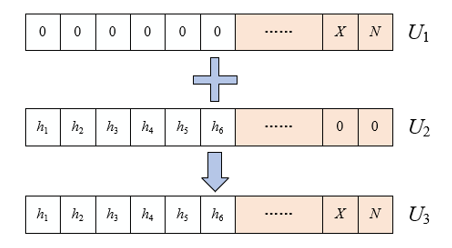Figure 6. Feature vector splicing

STEP 3: the eigenvalues of ZN×N were solved and normalized. After arranging the eigenvalues in descending order from large to small and the corresponding normalized eigenvectors in columns, the matrix EN×N was constructed. Then the first column of EN×N was the eigenvector corresponding to the maximum eigenvalue of ZN×N.

STEP 4: matrix CM×N=BM×N EN×N was obtained by multiplying BM×N and EN×N together. Feature vector splicing was completed according to Figure 6. Let u be the feature dimension after dimensionality reduction, then the first u columns CM×u of CM×N was u-dimensional feature matrix after dimensionality reduction.

STEP 5: L2 norm normalization was performed again for the first u columns of CM×u. For the normalized feature vector, its cosine similarity was equivalent to the Euclidean distance.

The dimension of each infrared thermal image after dimensionality reduction was (M, N). That is, M thermal feature descriptors with dimension N were extracted from each infrared thermal image.

4. Experimental Results and Analysis

Figure 7 shows the obtained thermal value information and the gray scale image of corresponding visual image. According to the corresponding relationship in the figure, the larger the gray scale value, the higher the temperature value of the area of visual image. The thermal map was mapped, with gray scale values as the index, and max and min were the maximum and minimum gray scale values, respectively. In order to better highlight the thermal part of visual image, the thermal value information of the image was classified and then incorporated into the feature descriptor, further generating multi-dimensional feature description vector for the infrared thermal image target area.

## 7.png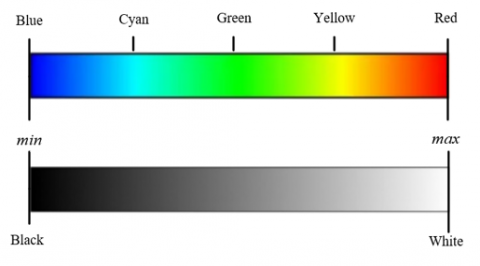Figure 7. Range of thermal value

The method in this paper was compared with other methods, such as CONGAS, CFNet, ATOM, SiamRPN++ and Fine-tuning CNN. Table 1 shows the evaluation results of target area extraction accuracy. Although the CONGAS algorithm, a traditional manual labeling feature method, achieved good results in the target area extraction of infrared thermal image, certain gap still remained between it and other deep learning based methods, such as CFNet, ATOM, SiamRPN++ and Fine-tuning CNN. Compared with these deep learning based methods, the method in this paper selected SegNet network as the semantic segmentation module in the target area extraction method of infrared thermal image, which achieved better extraction accuracy effect.

Table 1. Evaluation results of target area extraction accuracy

 Sample set No. CONGAS CFNet ATOM SiamRPN++ Fine-tuning CNN The method in this paper 1 63.4 81.4 85.3 86.4 86.1 88.6 2 61.2 79.3 84.6 84.6 70.3 87.1 3 63.4 87.3 93.4 82.4 93.6 94.1 4 56.7 85.4 87.6 82.3 90.6 93.4

Table 2. Comparison of average operation time of different methods

 Method Average operation time/s CONGAS 0.5213 CFNet 0.8164 ATOM 0.6154 SiamRPN++ 0.7456 Fine-tuning CNN 0.6741 The method in this paper 0.5134

A comparative analysis of the average operation time of six methods was conducted in order to further verify the effectiveness of the method used in this paper. According to Table 2, the average operation time of the method in this paper reduced 0.079 s compared with CONGAS algorithm, which was not large. However, compared with CFNet, ATOM, SiamRPN++ and Fine-tuning CNN, the average operation time of the method in this paper was lower, and a higher accuracy rate of target area extraction was obtained, thus verifying that the method in this paper was more practical.

Figure 8 shows the average recall ratio, average precision ratio, P-R curve and mAP curve, and compares the target area extraction accuracy of six methods. According to the figure, the method in this paper has better performance than other comparison methods in the above four aspects. When the number of sample images was low, the average precision ratio of the method used in this paper for target area extraction task of infrared thermal image was 97% and that of other methods was relatively low. As the number of sample images increased, all methods extracted the target area of infrared thermal image, which was less similar to the reference image, resulting in a decrease in the average precision ratio.

With the curve area enclosed by the P-R curve as the average retrieval accuracy mAP, the larger the area, the better the target area extraction effect of infrared thermal image. According to the figure, the method proposed in this paper has larger curve area and the highest position of mAP curve chart, indicating that the method proposed in this paper has better effect in extracting the infrared thermal image target area.

The extraction accuracy of target area of different targets in twelve different scenes was compared, and the method in this paper was compared with four deep learning based methods, namely, CFNet, ATOM, SiamRPN++ and Fine-tuning CNN. Table 3 shows the mAP comparison of target area extraction in twelve different scenes. According to the table, the method in this paper has obtained higher mAP values in target area extraction of different targets in twelve different scenes, further verifying that the method in this paper has better target area extraction performance.

Table 3. Comparison of target area extraction accuracy in twelve different scenes

 Scene CFNet ATOM SiamRPN++ Fine-tuning CNN The method in this paper 1 0.846541 0.814654 0.854647 0.846461 0.86135 2 0.764564 0.813135 0.854631 0.875164 0.946541 3 0.712516 0.854634 0.865461 0.748435 0.921316 4 0.644654 0.746349 0.813349 0.781324 0.821312 5 0.745496 0.761353 0.846136 0.821654 0.851313 6 0.815464 0.921464 0.913134 0.521251 0.915643 7 0.484646 0.52315 0.514674 0.613153 0.713251 8 0.915463 0.946461 0.946316 0.911651 0.965646 9 0.951466 0.971134 0.913164 0.915464 0.985163 10 0.834564 0.864521 0.841341 0.811653 0.871311 11 0.951464 0.964546 0.975138 0.944587 0.987212 12 0.947845 0.966347 0.941315 0.935156 0.972102

## 8a.png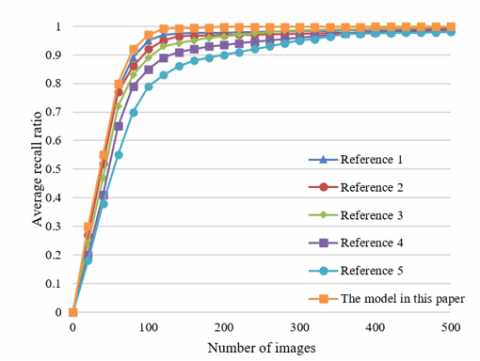a) Average recall ratio

## 9.png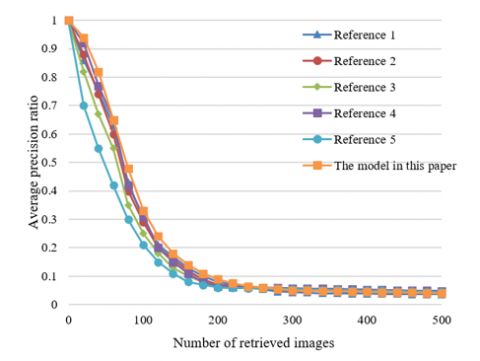b) Average precision ratio

## 10.png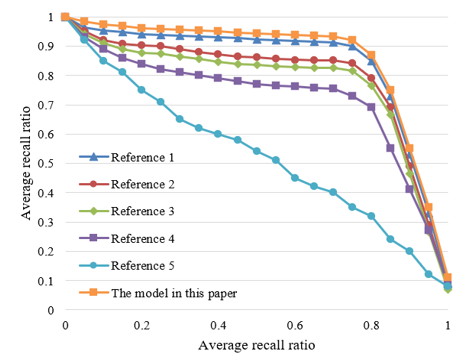c) P-R curve

## 8d.png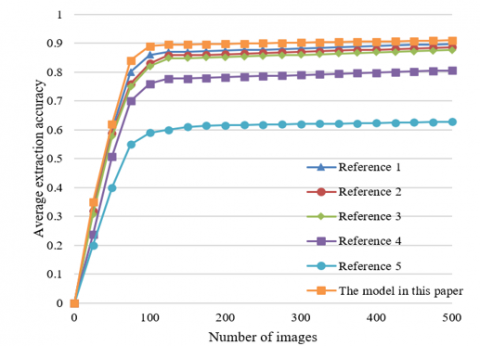d) mAP curve

Figure 8. Comparison chart of target area extraction accuracy

5. Conclusion

This paper did research on the target area extraction algorithm of infrared thermal image by combining target detection with matching correction. The second chapter introduced the feature matching algorithm based on grid motion statistics, and converted smoothness constraint of motion into statistics, thus replacing the number of extended feature points with the acquisition of features with better performance and filtering false image matching based on the number of other matching points in the neighborhood of statistical matching points. Based on the feature matching results obtained in the previous section, the third chapter proposed a method extracting infrared thermal image target area based on thermal feature descriptors, combining extracted thermal features with semantic attributes of each area in the image, thus distinguishing the subtle differences between the infrared thermal image sub-categories. This paper gave the evaluation results of target area extraction accuracy, by combining the thermal value information obtained in experiment with the gray scale image of corresponding visual image, and comparing the method proposed in this paper with other methods, namely, CONGAS, CFNet, ATOM, SiamRPN++ and Fine-tuning CNN. Then this paper made a comparative analysis of average operation time of six methods, which verified that the method in this paper was more practical. In addition, this paper gave the average recall ratio, average precision ratio, P-R curve and mAP curve, and compared the target area extraction accuracy of six methods, whose analysis showed that the target area extraction using the method in this paper had better effect. Finally, this paper compared the  target region extraction accuracy of different objects in twelve different scenes, which further verified that the method in this paper had good performance in target area extraction.

References

 Li, Z., Wang, L., Yu, J., Cheng, B., Hao, L., Jiang, S. (2019). Wide area remote sensing image on orbit target extraction and identification method. In 2019 IEEE International Conference on Signal, Information and Data Processing (ICSIDP), Chongqing, China, pp. 1-8. https://doi.org/10.1109/ICSIDP47821.2019.9173401

 Li, N., Wu, J. (2018). Research on methods of high coherent target extraction in urban area based on psinsar technology. International Archives of the Photogrammetry, Remote Sensing & Spatial Information Sciences, 42(3): 901-908. https://doi.org/10.5194/isprs-archives-XLII-3-901-2018

 Yang, J., Lu, J., Liu, X., Geng, Z. (2018). Fast imaging method based on target area extraction for wideband radar. In 2018 14th IEEE International Conference on Signal Processing (ICSP), Beijing, China, pp. 919-923. https://doi.org/10.1109/ICSP.2018.8652464

 Zou, B., Lu, D., Wu, Z., Qiao, Z.G. (2016). Urban-area extraction from polarimetric SAR image using combination of target decomposition and orientation angle. In Radar Sensor Technology XX, 9829: 536-542. https://doi.org/10.1117/12.2228689

 Han, L., Wu, T., Liu, Q., Liu, Z., Zhang, T. (2019). Extraction of target geological hazard areas in loess cover areas based on mixed total sieving algorithm. In Geo-informatics in Sustainable Ecosystem and Society: 6th International Conference, GSES 2018, Handan, China, pp. 208-214. https://doi.org/10.1007/978-981-13-7025-0_22

 Wu, W., Guo, H., Li, X. (2014). Urban area SAR image man-made target extraction based on the product model and the time–frequency analysis. IEEE Journal of Selected Topics in Applied Earth Observations and Remote Sensing, 8(3): 943-952. https://doi.org/10.1109/JSTARS.2014.2371064

 Yan, H., Wang, R., Li, F., Deng, Y., Liu, Y. (2012). Ground moving target extraction in a multichannel wide-area surveillance SAR/GMTI system via the relaxed PCP. IEEE Geoscience and Remote Sensing Letters, 10(3): 617-621. https://doi.org/10.1109/LGRS.2012.2216248

 Chen, Y.Q., Zhao, B.N., Chen, C., Zhao, B.B., Zhao, P.D. (2022). Identification of ore-finding targets using the anomaly components of ore-forming element associations extracted by SVD and PCA in the Jiaodong gold cluster area, Eastern China. Ore Geology Reviews, 144: 104866. https://doi.org/10.1016/j.oregeorev.2022.104866

 Wu, W., Guo, H., Li, X. (2013). Man-made target detection in urban areas based on a new azimuth stationarity extraction method. IEEE Journal of Selected Topics in Applied Earth Observations and Remote Sensing, 6(3): 1138-1146. https://doi.org/10.1109/JSTARS.2013.2243700

 Yan, H., Li, F., Robert, W., Zheng, M.J., Gao, C.G., Deng, Y.K. (2012). Moving targets extraction in multichannel wide-area surveillance system by exploiting sparse phase matrix. IET Radar, Sonar & Navigation, 6(9): 913-920. https://doi.org/10.1049/iet-rsn.2012.0067

 Deng, Y.K., Yan, H., Wang, R., Li, F., Ai, J.Q. (2012). A novel method to extract moving targets in the wide-area surveillance airborne SAR/GMTI system. In EUSAR 2012; 9th European Conference on Synthetic Aperture Radar Nuremberg, Germany, pp. 396-399.

 Yang, S., Sun, M., Lou, X., Yang, H., Zhou, H. (2023). An unpaired thermal infrared image translation method using GMA-CycleGAN. Remote Sensing, 15(3): 663. https://doi.org/10.3390/rs15030663

 Kirubakaran, V., Preethi, D.M.D., Arunachalam, U., Rao, Y.K., Gatasheh, M.K., Hoda, N., Anbese, E.M. (2022). Infrared thermal images of solar PV panels for fault identification using image processing technique. International Journal of Photoenergy, 2022: 6427076. https://doi.org/10.1155/2022/6427076

 Ninh, H., Thai, C., Hai, T.T. (2022). Deep learning-based infrared image deblurring. In Electro-optical and Infrared Systems: Technology and Applications XIX, 12271: 142-149. https://doi.org/10.1117/12.2636946

 Guei, A.C., Akhloufi, M.A. (2018). Deep generative adversarial networks for infrared image enhancement. In Thermosense: Thermal Infrared Applications XL, 10661: 37-48. https://doi.org/10.1117/12.2304875

 Wang, H., Cheng, C., Zhang, X., Sun, H. (2022). Towards high-quality thermal infrared image colorization via attention-based hierarchical network. Neurocomputing, 501: 318-327. https://doi.org/10.1016/j.neucom.2022.06.02

 Sathyan, N.M., Karthikeyan, S.R. (2022). Infrared thermal image enhancement in cold spot detection of condenser air ingress. Traitement du Signal, 39(1): 323-329.

 Chandra, S., AlMansoor, K., Chen, C., Shi, Y., Seo, H. (2022). Deep learning based infrared thermal image analysis of complex pavement defect conditions considering seasonal effect. Sensors, 22(23): 9365. https://doi.org/10.3390/s22239365

 Chen, Y., Cheng, L., Wu, H., Mo, F., Chen, Z. (2022). Infrared and visible image fusion based on iterative differential thermal information filter. Optics and Lasers in Engineering, 148: 106776. https://doi.org/10.1016/j.optlaseng.2021.106776

 Wang, L., Hu, W., Hu, Y. (2022). Study on the change law of red-green-blue values of infrared thermal image in the process of anthracite oxidation and spontaneous combustion. Frontiers in Materials, 9: 129. https://doi.org/10.3389/fmats.2022.865248

 Luo, F., Li, Y., Zeng, G., Peng, P., Wang, G., Li, Y. (2022). Thermal infrared image colorization for nighttime driving scenes with top-down guided attention. IEEE Transactions on Intelligent Transportation Systems, 23(9): 15808-15823. https://doi.org/10.1109/TITS.2022.3145476

 Gao, S., Ruan, Y., Hong, Q., Yin, D. (2022). Infrared thermal image fault detection based on YOLOV3-L. In 2022 IEEE International Conference on Artificial Intelligence and Computer Applications (ICAICA) Dalian, China, pp. 175-178. 10.1109/ICAICA54878.2022.9844534

 Domino, M., Borowska, M., Kozłowska, N., Zdrojkowski, Ł., Jasiński, T., Smyth, G., Maśko, M. (2021). Advances in thermal image analysis for the detection of pregnancy in horses using infrared thermography. Sensors, 22(1): 191. https://doi.org/10.3390/s22010191

 Yu, Z., Juanjuan, Z., Rui, L., Haidong, Z. (2021). Registration of thermal infrared image and visible image based on featured contour quadrilateral. Infrared and Laser Engineering, 50(S2): 20200520-1. https://doi.org/10.3788/IRLA20200520

 Akula, A., Ghosh, R., Kumar, S., Sardana, H.K. (2019). WignerMSER: Pseudo-wigner distribution enriched MSER feature detector for object recognition in thermal infrared images. IEEE Sensors Journal, 19(11): 4221-4228. https://doi.org/10.1109/JSEN.2019.2900268

 Zhang, Y., Tian, J., Yang, M., Yi, Y., Wang, Y., Zhang, Y. (2018). Screening of zero-value insulators infrared thermal image features based on binary logistic regression analysis. In 2018 2nd IEEE Conference on Energy Internet and Energy System Integration (EI2), Beijing, China, pp. 1-4. https://doi.org/10.1109/EI2.2018.8582434

 Lei, G., Yin, C., Huang, X., Dadras, S., Dadras, S. (2021). A decomposition multi-objective optimization method based on dynamic weight adjustment for infrared thermal image defect feature adaptive extraction method. In 2021 American Control Conference (ACC) New Orleans, LA, USA, pp. 4970-4975. https://doi.org/10.23919/ACC50511.2021.9483009